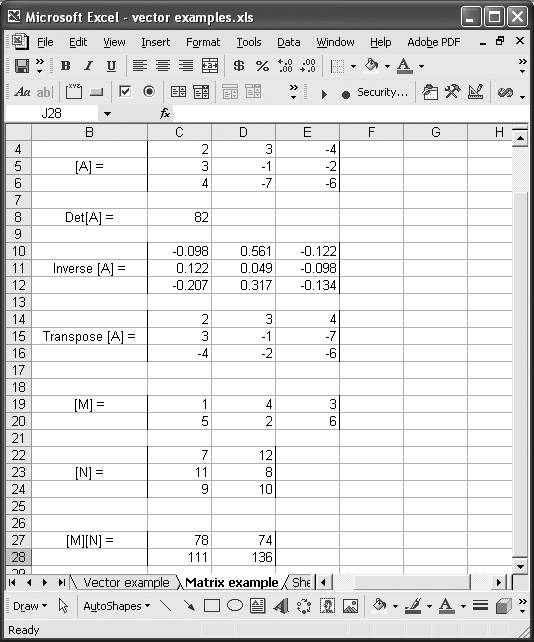# Manipulating Matrices

Problem

You need to manipulate matrices in your calculations and would like to know what support Excel offers for dealing with matrices.

Solution

Excel has several built-in matrix functions for computing matrix inverses and determinants and for multiplying or transposing matrices.

Discussion

Table 7-7 summarizes Excel's built-in matrix functions.

Table 7-7. Excel's built-in matrix functions

Function

Syntax

Description

MDETERM

=MDETERM(cell range)

Returns the determinant of the matrix argument, which must be a square matrix.

MINVERSE

=MINVERSE(cell range)

Returns the inverse of the matrix argument, which must be a square matrix.

MMULT

=MMULT(cell range 1, cell range 2)

Returns the matrix product of the two matrix arguments. The resulting matrix has the same number of rows as the first argument and the same number of columns as the second.

trANSPOSE

=trANSPOSE(cell range)

Returns the transpose of the matrix argument.

MDETERM returns a scalar, which means you enter the function as a normal function, by pressing Enter. The other three functions are array functions, which means you first have to select an appropriate number of cells, then type the function and press Ctrl-Shift-Enter to enter the function.

Figure 7-3 shows how these functions can be used in a spreadsheet.

Figure 7-3. Matrix functionsTo enter a matrix in a spreadsheet, just type the matrix elements in a series of cells as shown in Figure 7-3. Matrix A is a 3 x 3 matrix whose elements are contained in cells C4 through E6.

To compute the determinant of A, you can enter the formula =MDETERM(C4:E6). This formula was entered in cell C8 of the spreadsheet shown in Figure 7-3. The result is 82.

The inverse of A is shown in cells C10 to E12. To compute the inverse, select the cell range from C10 to E12, type the formula =MINVERSE(C4:E6), and press Ctrl-Shift-Enter.

You can use a similar procedure to compute the transpose of A, as shown in cells C14 to E16. The formula to enter is =trANSPOSE(C4:E6).

Cells C19 to E20 contain a 2 x 3 matrix, M. Another matrix, N, is contained in cells C22 to D24. N is a 3 x 2 matrix. To compute the matrix product MN, select a 2 x 2 range of cells and type the formula =MMULT(C19:E20,C22:D24). Press Ctrl-Shift-Enter to enter the formula. The results for this example are contained in cells C27 to D28.

Take a look at Recipe 9.4 for an example application of matrix operations.Excel Scientific and Engineering Cookbook (Cookbooks (OReilly))
ISBN: 0596008791
EAN: 2147483647
Year: N/A
Pages: 206
Authors: David M Bourg